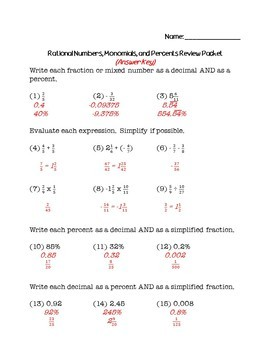# 8th Grade Math Review Packet BundleSubject
Resource Type
Product Rating
File Type

Compressed Zip File

2 MB|47 pages
Share
5 Products in this Bundle
5 products
1. This resource is a review packet for 8th grade math that includes 51 problems related to rational numbers, monomials, and percents. A full list of the topics included is below. An answer key is included. You can save by purchasing this as a part of a bundle that includes all five of my 8th grade ma
2. This resource is a review packet for 8th grade math that includes 34 problems related to writing equations, solving equations, writing inequalities, graphing inequalities, and simplifying algebraic expressions. A full list of the topics included is below. An answer key is included. You can save by
3. This resource is a review packet for 8th grade math that includes 25 problems related to angles, polygons, and the Pythagorean Theorem. A full list of the topics included is below. An answer key is included. You can save by purchasing this as a part of a bundle that includes all five of my 8th grad
4. This resource is a review packet for 8th grade math that includes 19 problems related to volume and surface area. A full list of the topics included is below. An answer key is included. You can save by purchasing this as a part of a bundle that includes all five of my 8th grade math review packets
5. This resource is a review packet for 8th grade math that includes 18 problems related to statistics and probability. A full list of the topics included is below. An answer key is included. You can save by purchasing this as a part of a bundle that includes all five of my 8th grade math review packe
Bundle Description
This bundle includes 5 different packets that cover a wide range of 8th grade math concepts. It includes a total of 147 problems. An answer key is included for all packets. Below is a summary of each packet and the specific topics included.

RATIONAL NUMBERS, MONOMIALS, and PERCENTS
(1) Changing fractions to decimals and percents
(2) Adding, subtracting, multiplying, and dividing fractions
(3) Changing percents to decimals and fractions
(4) Changing decimals to percents and fractions
(5) Percents problems
(6) Discount and sales tax
(7) Simple interest and compound interest
(8) Evaluating with exponents
(9) Simplifying with exponents
(10) Negative exponents
(11) Writing numbers in scientific notation and standard form
(12) Computations in scientific notation
(13) Estimating roots

EQUATIONS AND INEQUALITIES
(1) Solving one-step equations
(2) Solving two-step equations
(3) Solving multi-step equations (with variable on both sides)
(4) Writing equations
(5) Rewriting expressions using the Distributive Property
(6) Simplifying algebraic expressions
(7) Graphing inequalities
(8) Solving one-step inequalities
(9) Solving two-step inequalities
(10) Solving multi-step inequalities (with variable on both sides)
(11) Writing inequalities

ANGLES, POLYGONS, AND THE PYTHAGOREAN THEOREM
(1) Using angle relationships to find missing angles
(2) Identifying complementary and supplementary angles
(3) Identifying alternate interior, alternate exterior, and corresponding angles
(4) Finding missing angles in triangles and quadrilaterals
(5) Finding the measure of an interior angle of regular polygons
(6) Determining whether two polygons are similar figures
(7) Finding the tangent of an acute angle in a right triangle
(8) Using the Pythagorean Theorem to find missing side lengths of right triangles
(9) Using the Distance Formula to find the distance between two points
(10) Using properties of special right triangles

VOLUME AND SURFACE AREA
(1) Volume and surface area of rectangular prisms
(2) Volume and surface area of triangular prisms
(3) Volume and surface area of cylinders
(4) Volume and surface area of pyramids
(5) Volume and surface area of cones
(6) Volume and surface area of composite figures

STATISTICS AND PROBABILITY
(1) Finding the mean, median, mode, and range of a set of data
(2) Creating box-and-whisker plots
(3) Permutations
(4) Combinations
(5) Probability of Independent Events
(6) Probability of Dependent Events

You can get the majority of my 6th grade math and 8th grade math products at a large discount by purchasing them as a part of the following bundles. This review packet IS NOT a part of either mega bundle.

Math Mega Bundle (6th Grade Math)
Math Mega Bundle (8th Grade Math)

Also be sure to check out my Angle Relationship Task Cards for middle school math (not included in the Mega Bundles)!

Total Pages
47 pages
Included
Teaching Duration
N/A
Report this Resource
Reported resources will be reviewed by our team. Report this resource to let us know if this resource violates TpT’s content guidelines.